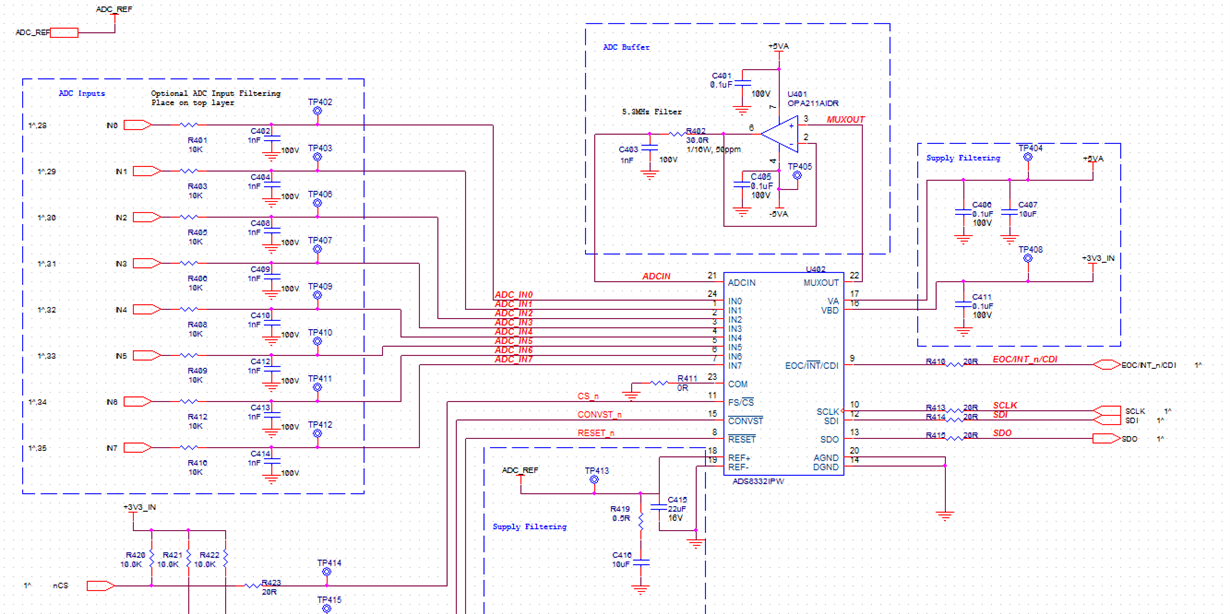If you have a related question, please click the "Ask a related question" button in the top right corner. The newly created question will be automatically linked to this question.

Hi,

I am using two ADS8332 configured in daisy chain mode to provide a total of 16 analog readings. The ADC voltage conversions are about 20% less than the expected value.

The ADS8332 is configured as follows:VA=5V, VBD=3.3V, VREF=4.096V. There is a buffer with a 5.3 MHz filter to drive the ADC Pin

I've taken some DC measurements when the voltage at IN1 is 1V:

• IN1: 0.986V
• VREF = 4.09505
• VA=5.043
• VBD=3.287

In one case, the IN1 voltage is expected to be 1V, however the conversion result is 0.78V. (a count of 12335 decimal)

I took some scope measurements of the ADC IN pin during conversion. The green trace is the unfiltered OPA211 output, the yellow trace is at the ADCIN pin.

The figure below shows ADCIN as the mux is switching sequentially from I0 through IN7.Thanks,

Igor

• Hi Igor

Input series resistor on each input channel  is 10kOhms. Is it application need. If not then can you reduce this value and see the performance

OPA211 has input impedance of around 20kOhm and this might be reducing the analog input voltage seen by ADCIN. Also OPA211 has input bias current of 60nA (typical value) that would introduce error of around 0.6mV too

If you can not reduce input series resistor value of 10k then you can use OPA209. It has comparatively higher input impedance (almost 10x of OPA211) and bias current of 1nA (typical).

Also for full scale input voltage (0 toVref) you have to increase Va to 5.5 (Please see input voltage range of OPA211/OPA209)

Thanks & Regards

Abhijeet

• Hi Abhijeet,

Is the low input impedance of the OPA211 the reason the rise time of ADCIN is slower than expected? The output filter on the OPA211 should have a rise time of 70ns, but I am seeing a rise time greater than 5us in the yellow trace above. The green trace is the output of the OPA211 and it is not slew rate limited.

For the increased Va, do you mean increase the Va of the ADS8332 or increase the supply rails of the OPA211/209

Will the using the OPA209 solve the acquisition error or will it just improve the offset error?

Thanks!

• Hi Igor

OPA211 input impedance may not be causing rise time delay at ADCIN. As you are seeing different voltage at ADCIN I wanted to make sure if that is caused by amplifier stage.

To ckeck this you can short  series resistor 10k on input channel and repeat the test to see if you get right code. If this solves the problem then we need to look at OPA211 stage

If this does not solve the problem then we have to look for other possibilities.

when I say Va=5.5V, I mean OPA211 supply voltage.

Thanks & Regards

Abhijeet